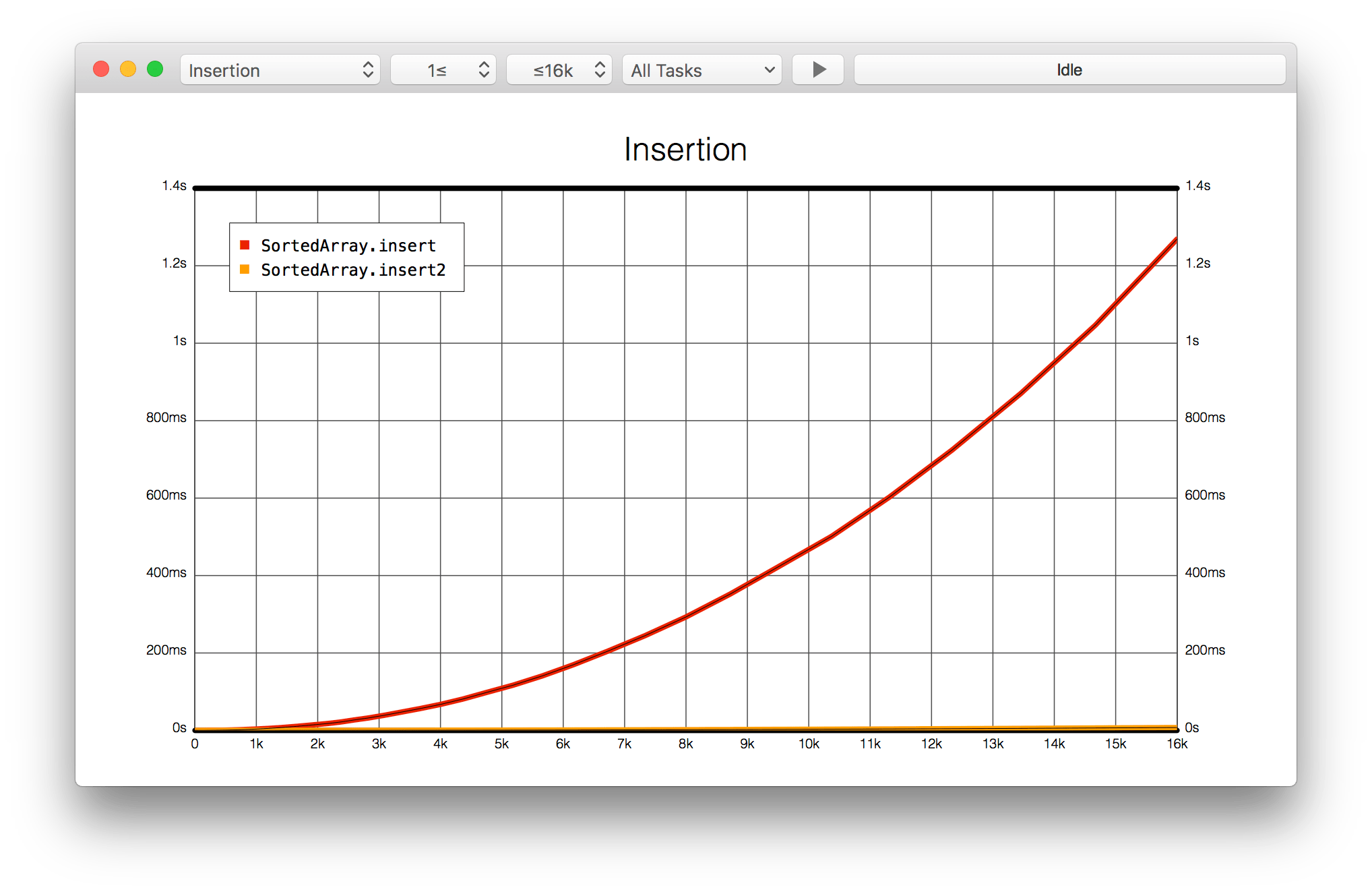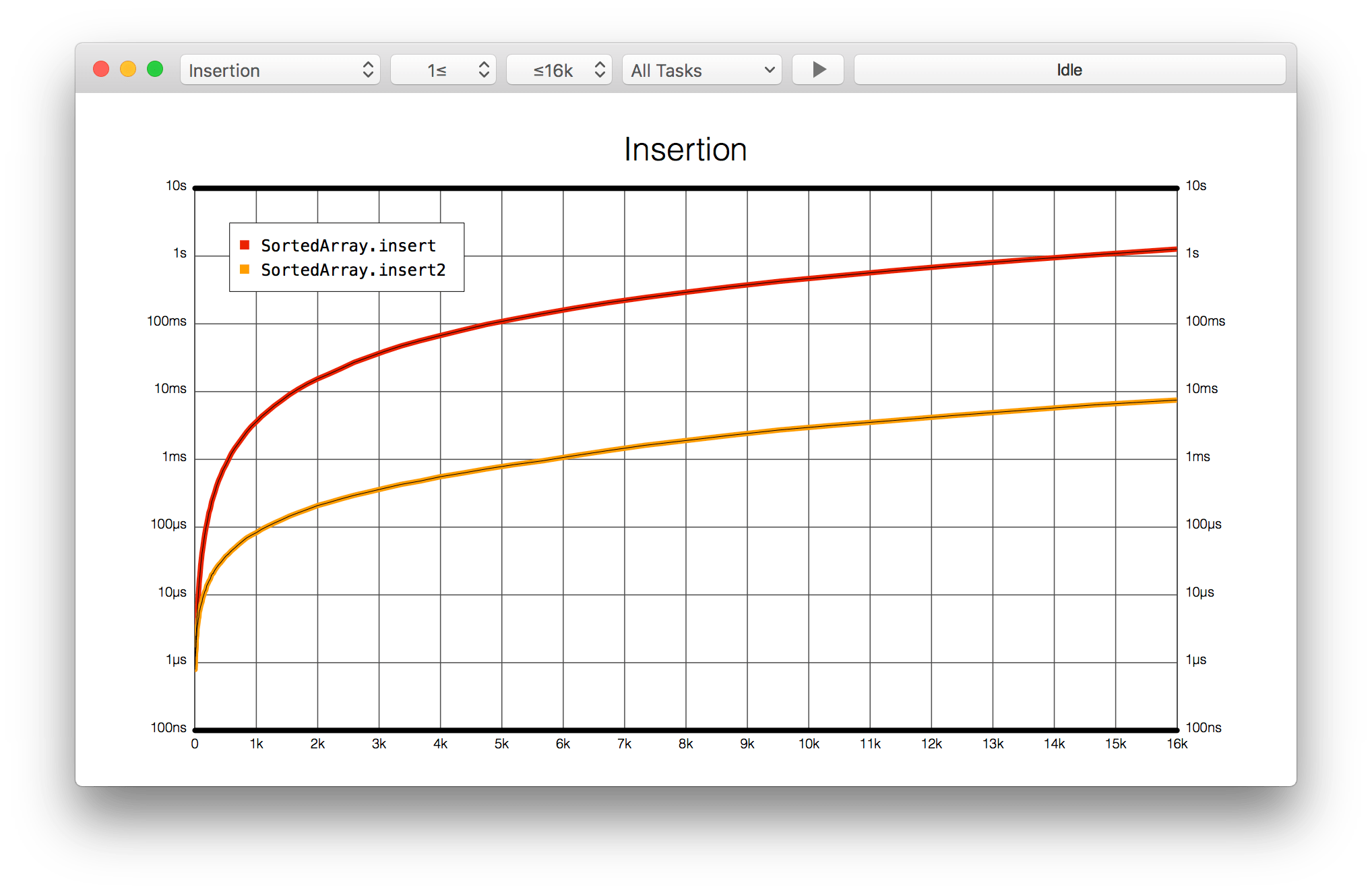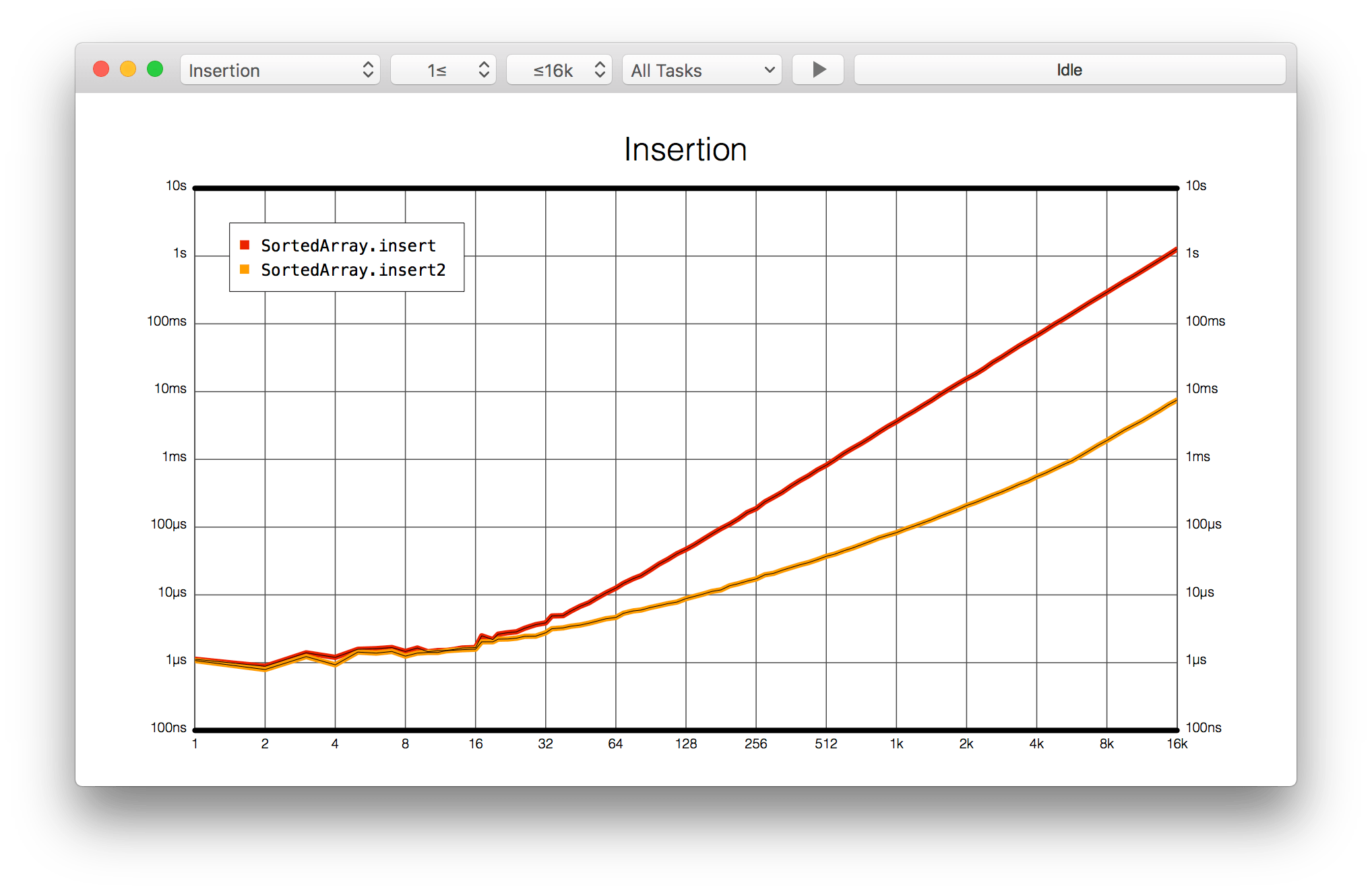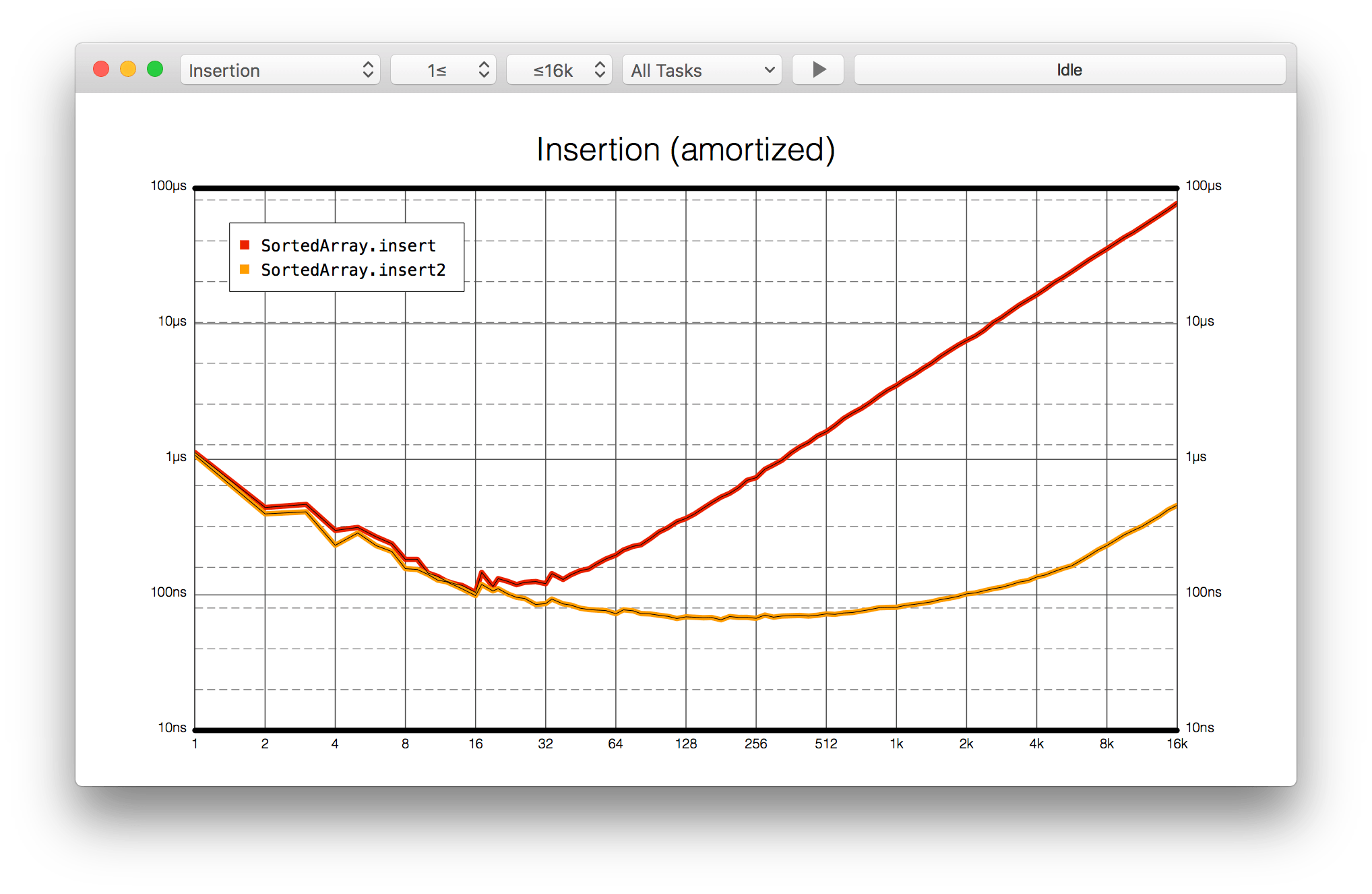Swift Talk # 55

## Sorted Arrays with Binary Search

with special guest Károly Lőrentey

### This episode is freely available thanks to the support of our subscribers

Together with Károly, we improve our sorted array implementation using binary search. We benchmark both implementations to learn about their real-world performance.

00:06 Today we meet up with Károly, who wrote a book called Optimizing Collections.

00:54 He'll help us optimize our `SortedArray` type, which we wrote in episode #35. This simplified version is constrained to work with `Comparable` elements:

``````import Foundation

struct SortedArray<Element: Comparable> {
var elements: [Element]

init<S: Sequence>(unsorted: S) where S.Iterator.Element == Element {
elements = unsorted.sorted()
}

mutating func insert(_ element: Element) {
elements.append(element)
elements.sort()
}
}
``````

## Optimizing insert

01:24 The `insert` method appends the new element and sorts the entire array again. By making the method a little bit more complex, we can improve the execution time.

01:52 We know the `elements` array is already sorted, so there's no reason to sort the entire array after inserting one new element. Instead, we can find the correct position at which to insert the new element.

02:28 One way of finding the correct position is by implementing binary search, which works by iterating over a range beginning with the entire array and splitting the range in half until it's narrowed down to one position in the array.

03:01 We define the range's initial bounds:

``````mutating func insert2(_ element: Element) {
var start = elements.startIndex
var end = elements.endIndex
while start < end {

}

}
``````

03:45 In the while loop, we could find the middle index by calculating it, like so:

``````let middle = (start + end) / 2
``````

04:01 The problem is that the addition might overflow if the array is huge, thereby crashing the application. We avoid this by adding half the distance to the `start` index:

``````let middle = start + (end - start) / 2
``````

05:01 Using the middle index, we can compare the middle element and the element that's to be inserted. If the middle element is less than the new element, we know that all elements of the first half of the array are less than the new element. In such a case, we can eliminate the first half and look at the second half. We update the `start` index accordingly:

``````if elements[middle] < element {
start = middle + 1
}
``````

05:48 Because we checked that the middle element is less than the new element, we can update `start` to be the index after the middle index.

06:19 Otherwise, if the element is less than or equal to the middle element, we have to continue with the left half. In that case, we update the `end` index:

``````if elements[middle] < element {
start = middle + 1
} else {
end = middle
}
``````

06:58 After the while loop, the `start` and `end` indices should be equal. We add an assertion for this:

``````assert(start == end)
``````

07:33 We've found the position at which to insert the new element. This completes the method:

``````mutating func insert2(_ element: Element) {
var start = elements.startIndex
var end = elements.endIndex
while start < end {
let middle = start + (end - start) / 2
if elements[middle] < element {
start = middle + 1
} else {
end = middle
}
}
assert(start == end)
elements.insert(element, at: start)
}
``````

07:54 Now we check our new insertion method:

``````var test = SortedArray<Int>(unsorted: [])
test.insert2(5)
test.insert2(4)
test.insert2(6)
// test.elements: [4, 5, 6]
``````

## Benchmarking

08:43 To test if the new implementation is faster, we can use a benchmarking application Károly wrote, called Attabench.

09:32 We prepared an empty benchmark, to which we'll add some tasks to measure:

``````import Foundation
import BenchmarkingTools

func randomArrayGenerator(_ size: Int) -> [Int] {
var values: [Int] = Array(0 ..< size)
values.shuffle()
return values
}

public func generateBenchmarks() -> [BenchmarkProtocol] {
return []
}

func sampleBenchmark() -> BenchmarkProtocol {
let benchmark = Benchmark(title: "Insertion", inputGenerator: randomArrayGenerator)
// ...
return benchmark
}

func containsBenchmark() -> BenchmarkProtocol {
let benchmark = Benchmark(title: "Contains", inputGenerator: randomArrayGenerator)
// ...
return benchmark
}
``````

10:03 We can add a task with a title and a closure containing code to be measured. The closure will receive an array of random integers. We add tasks for `insert` and `insert2` in order to compare the two implementations. In both cases, we start out with an empty `SortedArray` and loop through the random input array to insert each value:

``````func sampleBenchmark() -> BenchmarkProtocol {
let benchmark = Benchmark(title: "Insertion", inputGenerator: randomArrayGenerator)

var array = SortedArray<Int>(unsorted: [])
for value in input {
array.insert(value)
}
}

var array = SortedArray<Int>(unsorted: [])
for value in input {
array.insert2(value)
}
}

return benchmark
}
``````

12:14 In order for the application to find this benchmark, we have to add it to the return value of `generateBenchmarks()`:

``````public func generateBenchmarks() -> [BenchmarkProtocol] {
return [
sampleBenchmark()
]
}
``````

12:32 When we run this, the macOS application launches and we can find the benchmark tasks in its menu. After starting the execution, a graph is plotted showing measurements of the executed tasks, and we see a big difference between the two insertion methods. The slower `insert` task's graph looks like a parabola, but it's hard to see the shape of `insert2`, which almost appears as constant time:13:40 By switching the vertical time axis to a logarithmic scale, we see that the two tasks have a similar shape. But now we notice we have the same problem on the horizontal axis — it's hard to tell what's happening at the lower values on the left:14:41 The ideal chart for benchmarks has both axes on a logarithmic scale, so we can properly see how the tasks compare and perhaps detect patterns that aren't normally visible. Because a logarithmic plot allocates the same amount of space to each order of magnitude, it's easy to see at a glance how an algorithm behaves across the board:15:17 Currently, the graph shows the overall execution time for our benchmarks, but we're more interested in the average time it takes to do a single insertion. Fortunately, the benchmark tool offers a way to divide the execution time by the size of the array. This gives us an amortized chart, which displays the average time for a single insertion, plotted against array size:16:08 Now the graph gets interesting. When we continue the benchmark, we can tell that our new implementation of `insert2` is about 200 times faster than the original `insert`. That's quite impressive for a small modification of our code.

## Optimizing contains

17:11 We can also try to make `contains` faster. We move some of the code into a utility method we can reuse:

``````struct SortedArray<Element: Comparable> {
// ...

func index(for element: Element) -> Int {
var start = elements.startIndex
var end = elements.endIndex
while start < end {
let middle = start + (end - start) / 2
if elements[middle] < element {
start = middle + 1
} else {
end = middle
}
}
assert(start == end)
return start
}

mutating func insert2(_ element: Element) {
elements.insert(element, at: index(for: element))
}
}
``````

18:48 To write `contains2`, we can use `index(for:)` to check whether the element already exists at the index where it belongs. We should check that the returned index is not the array's `endIndex`. Otherwise, we'd get an out-of-bounds exception by using subscript:

``````func contains2(element: Element) -> Bool {
let index = self.index(for: element)
guard index < elements.endIndex else { return false }
return self[index] == element
}
``````

19:56 We can benchmark this method too — except this time, we have to start with a populated array before we can measure the execution of the `contains` method. To add the task, we use a different method in which we have to return a closure that takes a timer and uses it to measure a specific block of code. This way, we can put the setup of the `SortedArray` outside the measured code:

``````func containsBenchmark() -> BenchmarkProtocol {
let benchmark = Benchmark(title: "Contains", inputGenerator: randomArrayGenerator)

let array = SortedArray<Int>(unsorted: input)
return { timer in
timer.measure {
for value in input {
if !array.contains(value) { fatalError() }
}
}
}
}

let array = SortedArray<Int>(unsorted: input)
return { timer in
timer.measure {
for value in input {
if !array.contains2(element: value) { fatalError() }
}
}
}
}

return benchmark
}
``````

24:08 We add the new benchmark to the list of available benchmarks:

``````public func generateBenchmarks() -> [BenchmarkProtocol] {
return [
sampleBenchmark(),
containsBenchmark()
]
}
``````

24:19 We run the application and choose the "Contains" benchmark. Then we see the amortized chart:24:39 It appears that the original implementation of `contains` — which enumerates and compares each element — is faster for small arrays than our new version is. That's probably because the CPU can prefetch values when iterating over a continuous buffer. Besides, the algorithm of `contains` itself is much simpler, whereas the more complicated `contains2` adds some overhead by doing comparisons. However, the time difference is very small: in the worst case, with an array of around 16 elements, the difference in execution time of the two methods is a couple dozen nanoseconds.

26:37 There's a lot more to do with collection optimization. We'll continue exploring in a future episode.

### Resources

• #### Playground

Written in Swift 3.1

See All
Experiments

### Git Under the Hood: Blobs

Episode 357 · May 26

SwiftUI

### Connecting Lines with Anchors (Part 3)

Episode 356 · May 19

SwiftUI

### Connecting Lines with Anchors (Part 2)

Episode 355 · May 12

SwiftUI

### Connecting Lines with Anchors (Part 1)

Episode 354 · May 05

SwiftUI

### Reimplementing Anchors: Transforms

Episode 353 · Apr 28

SwiftUI

### Reimplementing Anchors: Points

Episode 352 · Apr 21

SwiftUI

### Reimplementing Anchors: Bounds

Episode 351 · Apr 14

SwiftUI

### Flow Layout Alignment

Episode 350 · Apr 07

Unlock Full Access

## Subscribe to Swift Talk

• ### Watch All Episodes

A new episode every week